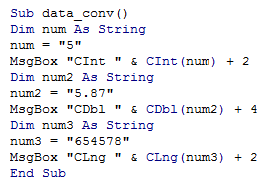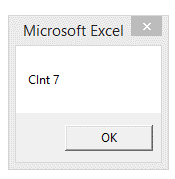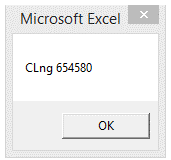## Vba datatype conversion in Excel

This topic is of much imporatnce and is most often used feature of VBA in Excel.

This is because, Excel sheet can store data in both text and numbers irrespective of the type of data.

So when a mathematical calculation has to be performed with some numbers that are stored as text,

the numbers has to be converted to correct datatype, else the program will return an error.

This is where the type conversion comes into the picture. The same is true for conversion from number to text.

The keywords associated with conversion are Cint,CStr,CDbl and Cdate

The following examples illustrates these

1. Sub data_conv()
2. Dim num As String
3. num = "5"
4. MsgBox "CInt " & CInt(num) + 2
5. Dim num2 As String
6. num2 = "5.87"
7. MsgBox "CDbl " & CDbl(num2) + 4
8. Dim num3 As String
9. num3 = "654578"
10. MsgBox "CLng " & CLng(num3) + 2
11. End Sub

From the above example, it is seen that, the numbers are stored as string which are converted to corresponding numerical type before performing mathematical calculation.

A glimpse of the editor screenshot is shown belowThe output is shown below